### The Atlantis cubits and stade resolved by high resolution satellite images of irrigation canals in the Altiplano, Bolivia.

Plato in his story of Atlantis relates that the plain was covered by a system of parallel canals at intervals of 100 "stades" with allotments whose size was 10 x 10 stades.

Study of the ringed mountain at Pampa Aullaags suggests that this stade might have been a unit of 165 feet (50.292 metres) and study of ancient canals of the Olmecs/Mayas in the Tabasco region of Mexico show regular blocks of irrigation canals in squares of 1650 feet, which would be similar to the 10 x 10 stade allotments described by Plato.

The 165 foot stade was a Sumerian measurement unit and consisted of 100 Sumerian cubits of 19.8" so each allotment of 10 stades had a side of 1,000 cubits.

In order to further confirm the use of Sumerian stades and cubits, this page examines some of the parallel canals found on the Bolivian Altiplano to the south of Oruro and it seems that some of the canals were set out in fixed distances apart, such as 300 cubits which is 3 stades of 165 feet.

The area is sparsley inhabted today but attempts are being made to re-introduce agriculture to the area and in many instances the old canals are being re-used today.above, these adjacent plots on the Altiplano are 1650 and 1980 feet long respectively, making the one on the left equal to 1000 cubits and the one on the right 1200 cubits therefore equal in length to "standard" Atlantis plot and the "warriors plot".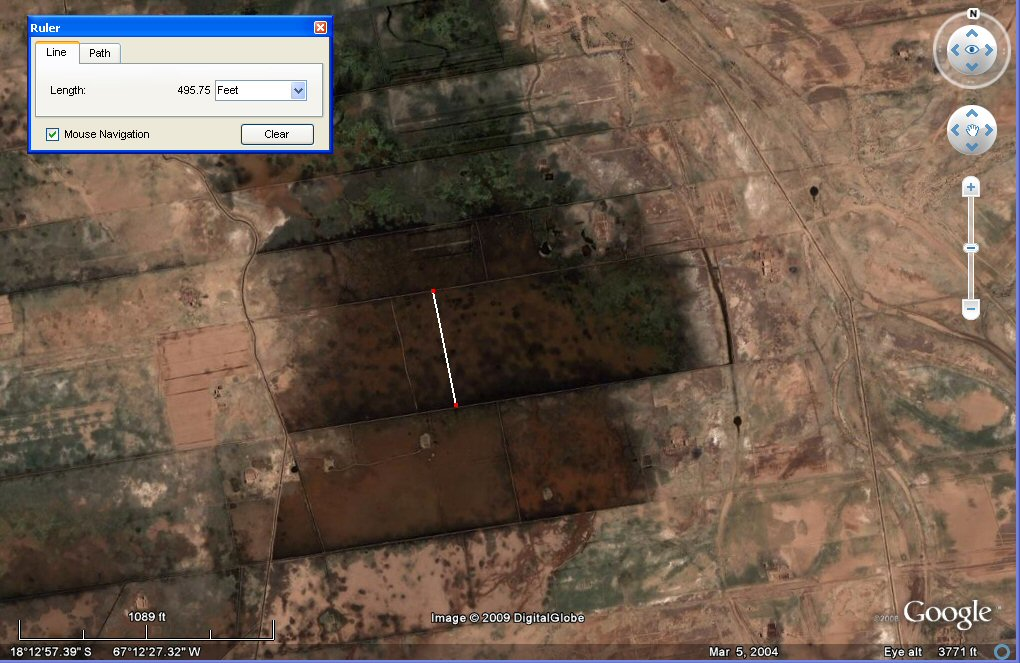above, image 21, intended distance 495 feet is 300 cubits of 19.8"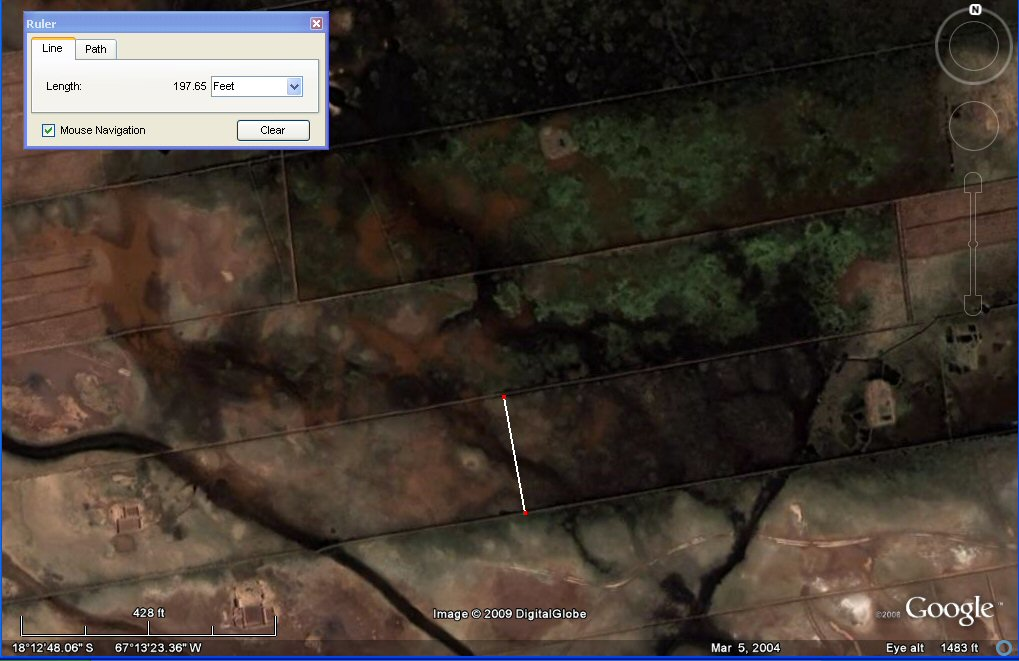above, image 22, intended distance 198 feet is 120 cubits of 19.8"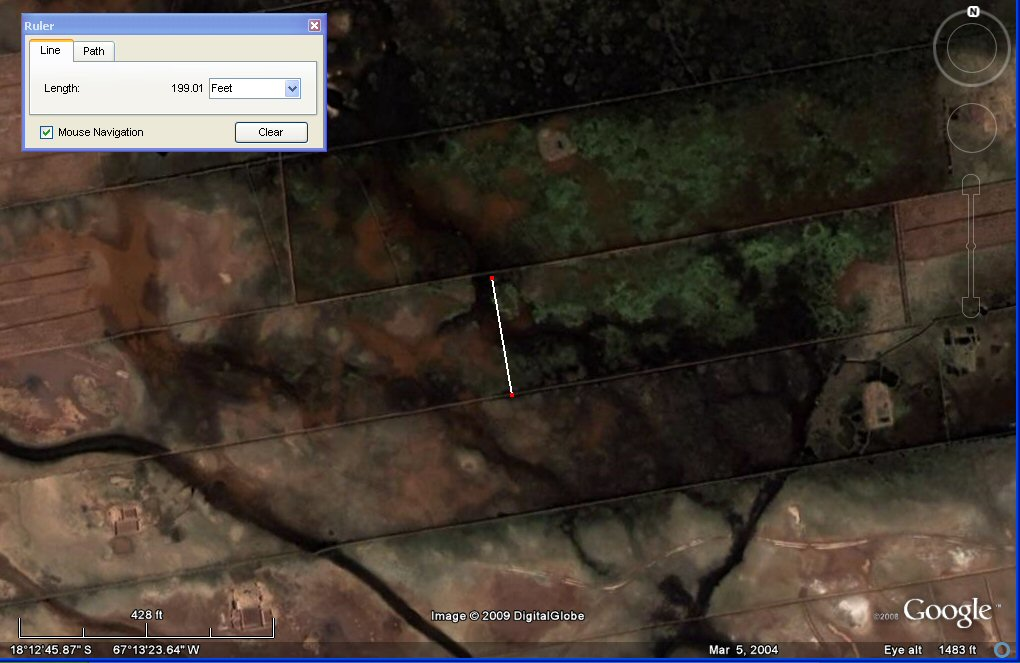above, image 23, adjacent plot, intended distance 198 feet is 120 cubits of 19.8"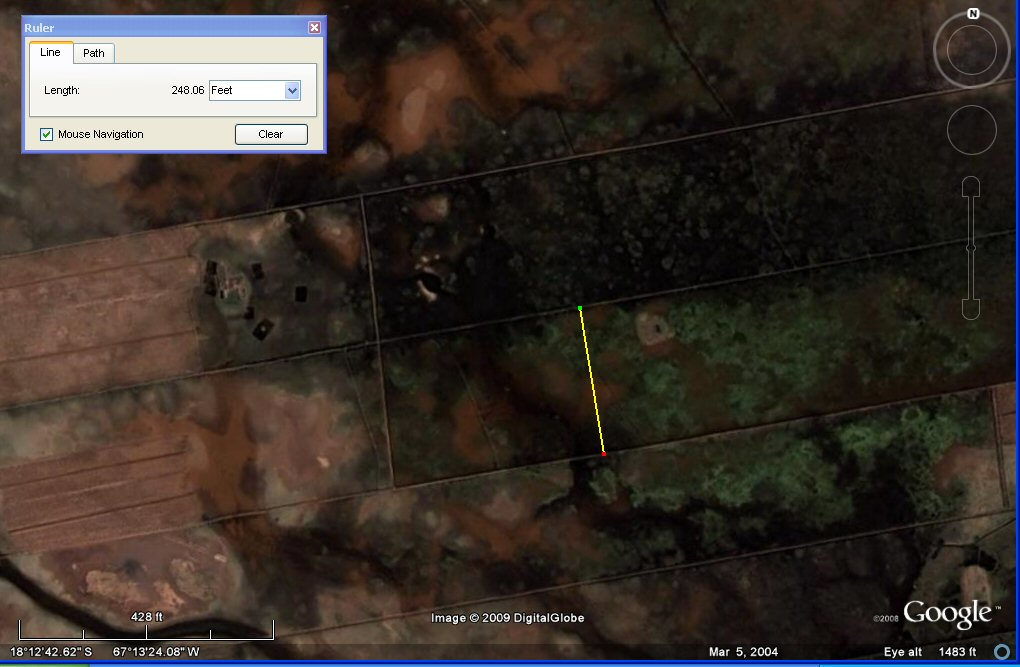above, image 24, intended distance 247.5 feet is 150 cubits of 19.8"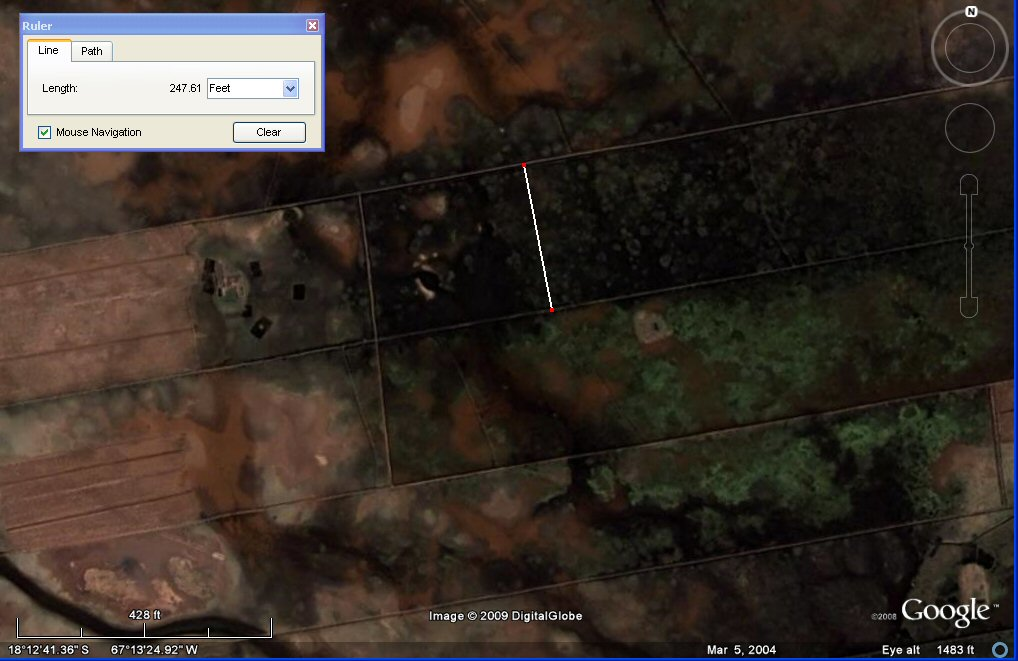above, image 25, adjacent plot, intended distance 247.5 feet is 150 cubits of 19.8"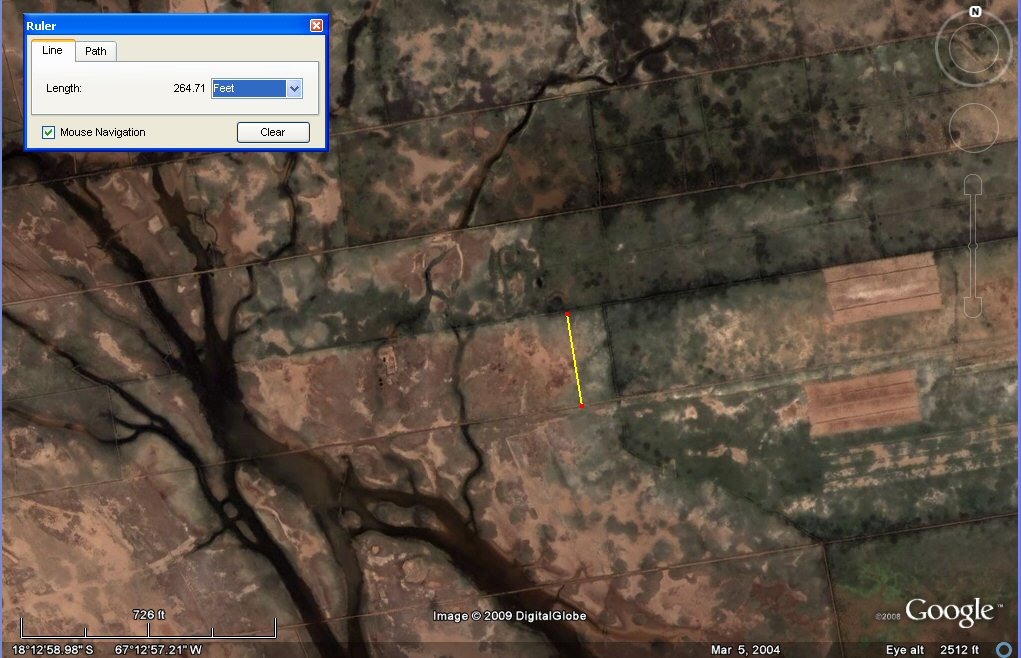above, image 26, intended distance 264 feet is 160 cubits of 19.8"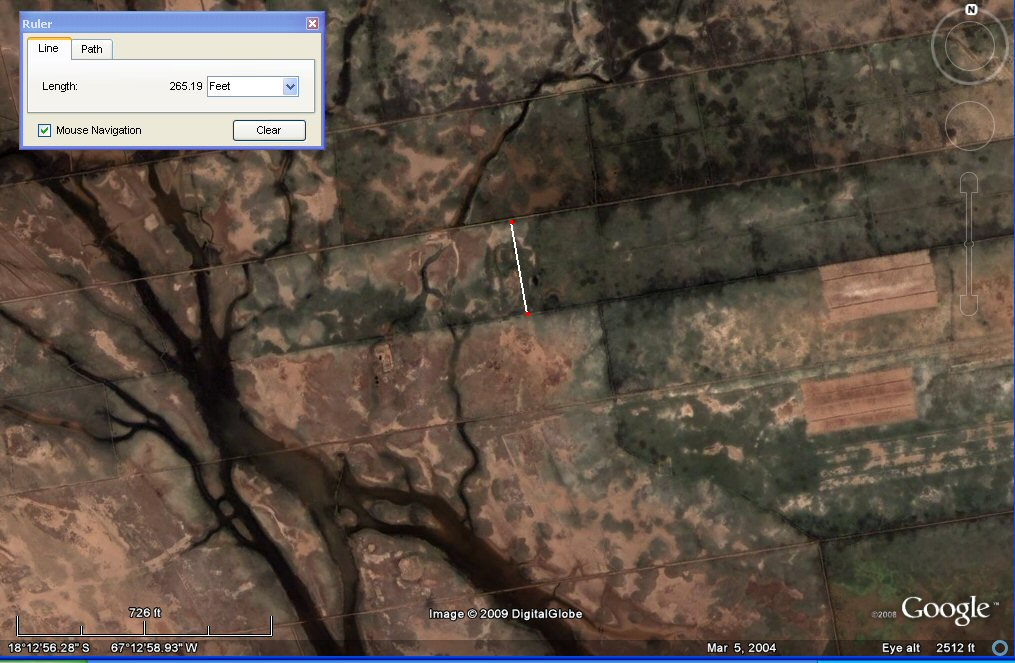above, image 27, adjacent plot, intended distance 264 feet is 160 cubits of 19.8"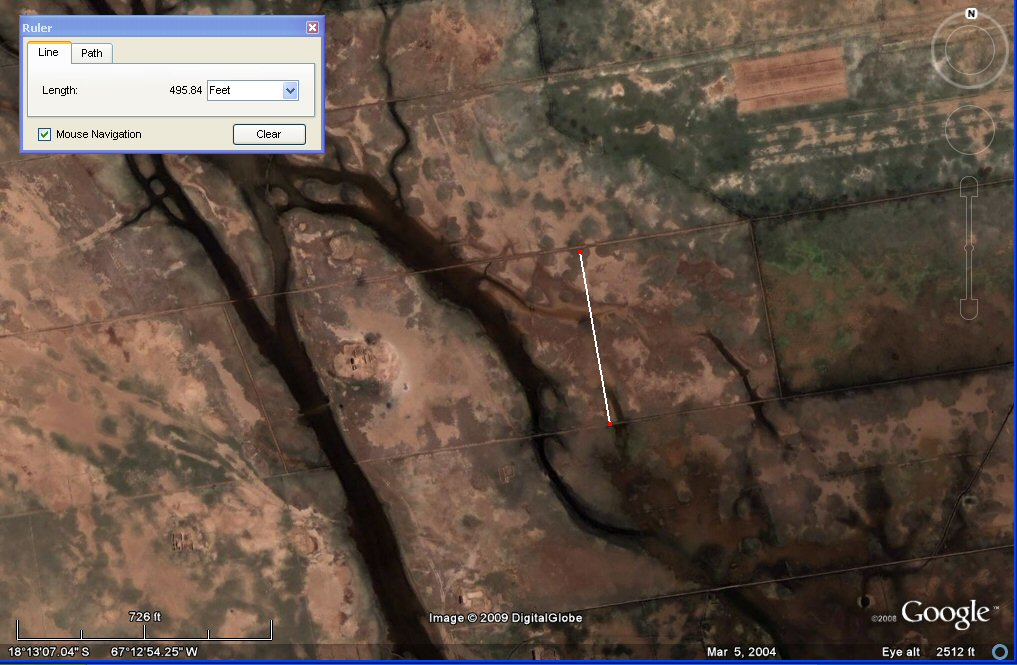above, image 29, intended distance 495 feet is 300 cubits of 19.8" which is 3 stades of 165 feet.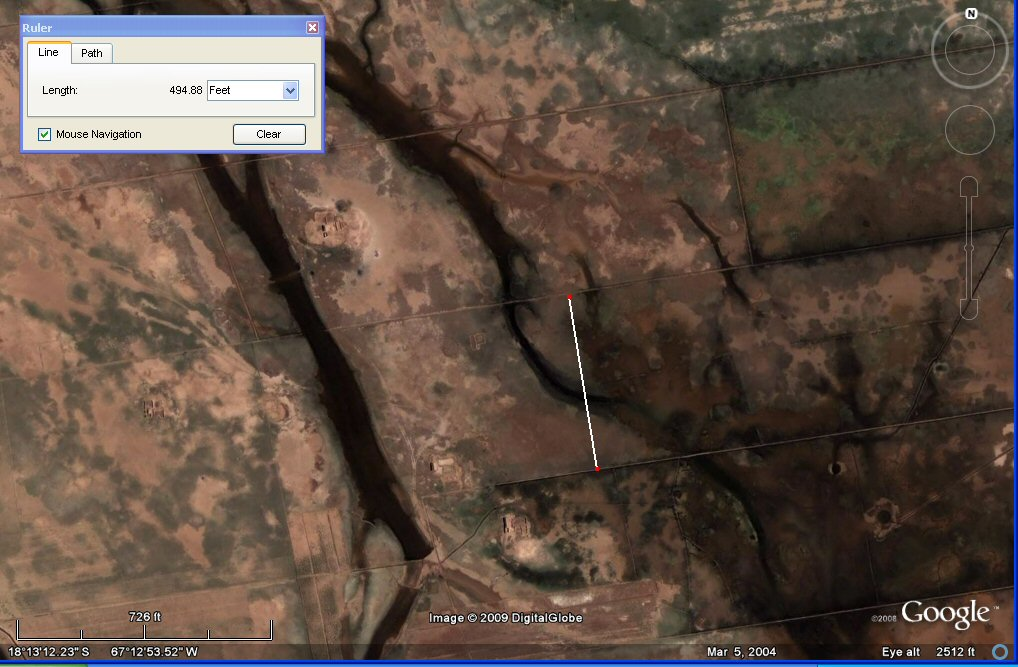above, image 30, adjacent plot, intended distance 495 feet is 300 cubits of 19.8" which is 3 stades of 165ft.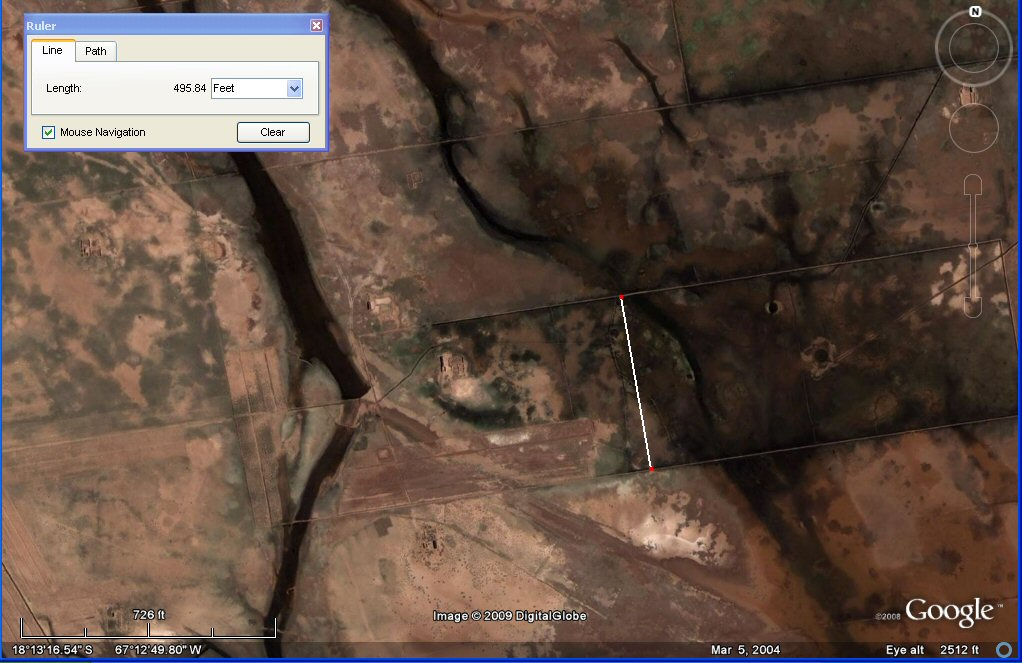above, image 31, the next adjacent plot to the south, intended distance 495 feet is 300 cubits of 19.8"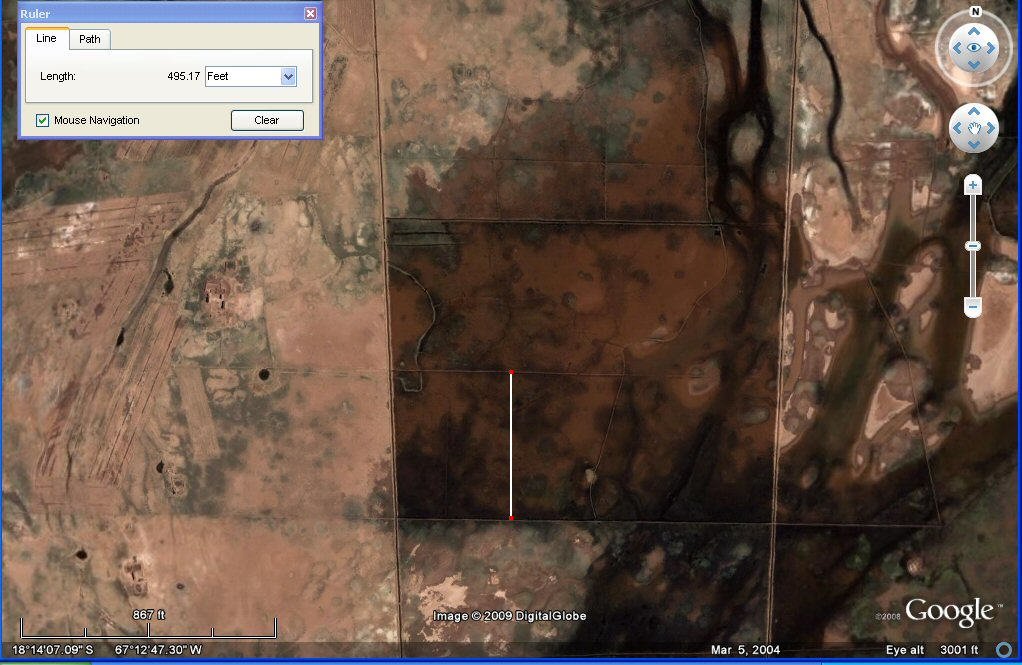above, image 34, intended distance 495 feet is 300 cubits of 19.8" which is 3 stades of 165 feet.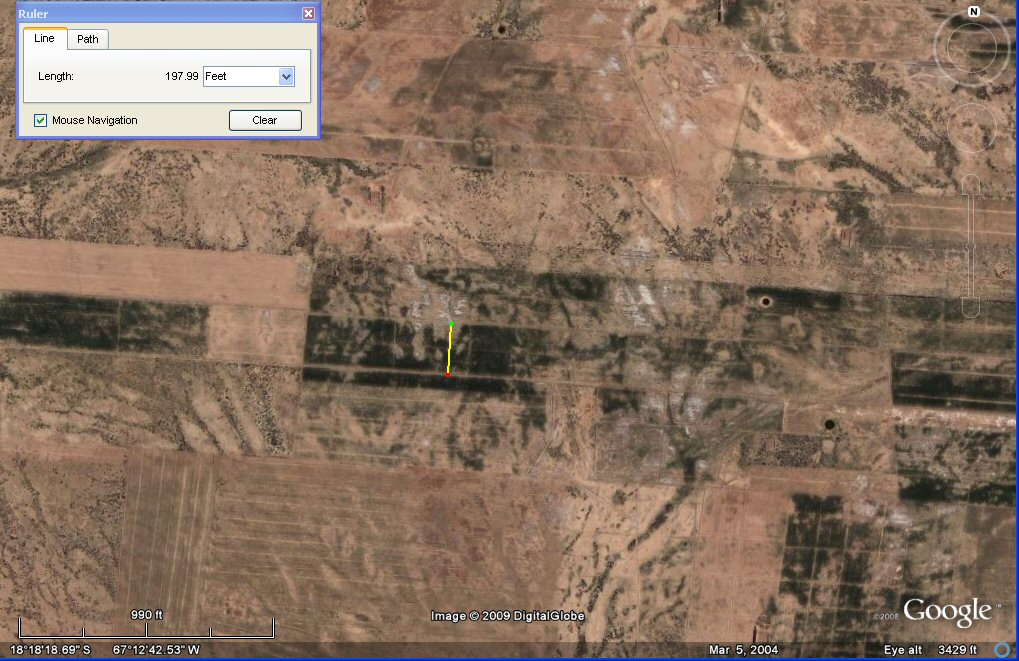above, image 37, intended distance 198 feet is 120 cubits of 19.8"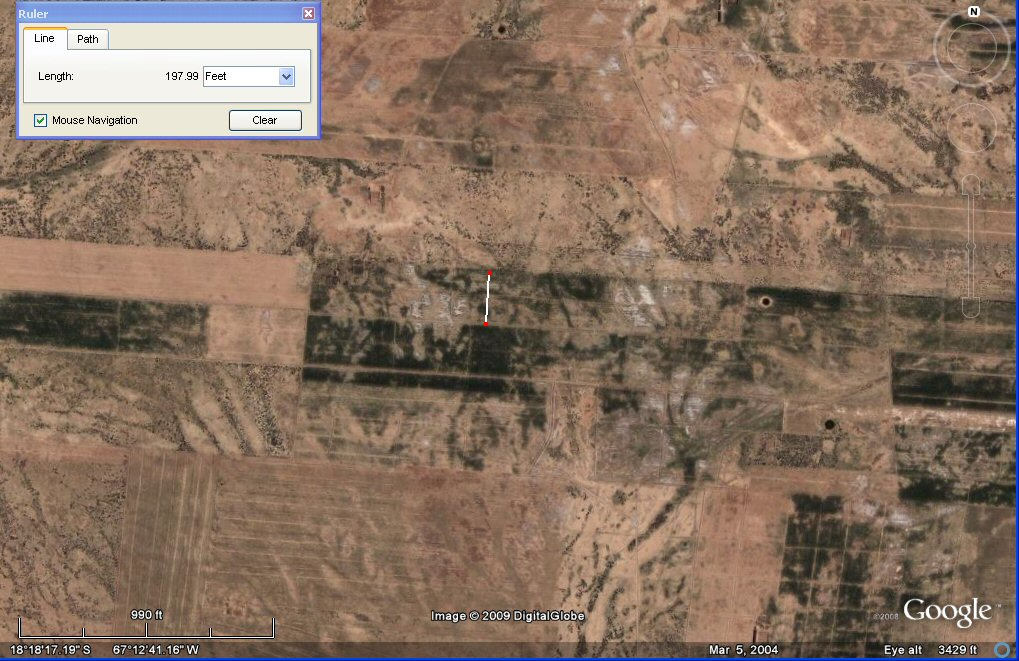above, image 30, adjacent plot, intended distance 198 feet is 120 cubits of 19.8"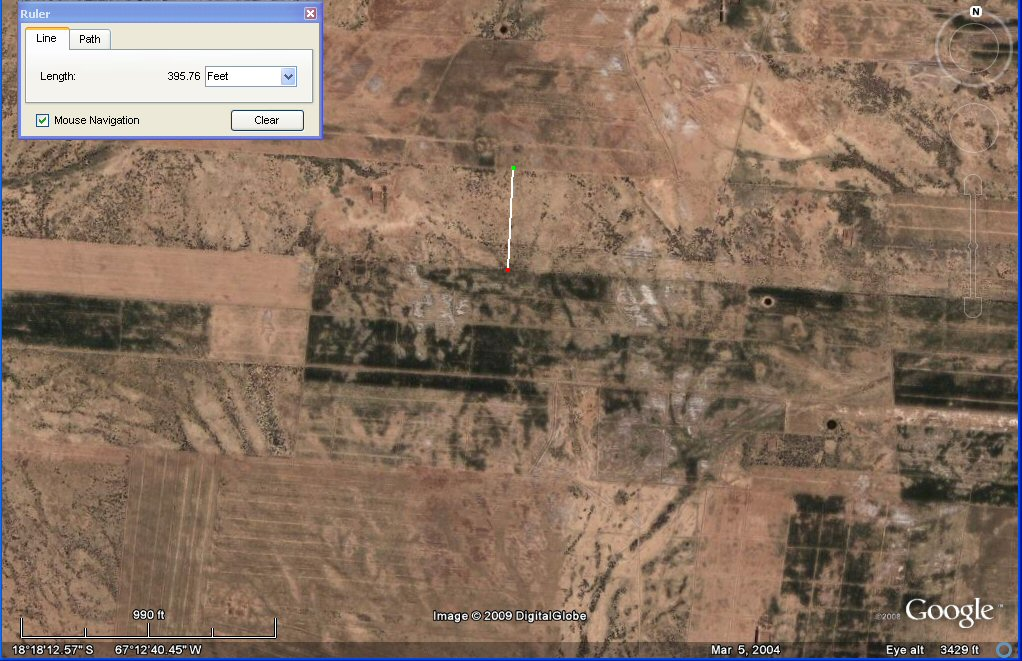above, image 39, intended distance 396 feet is 240 cubits of 19.8"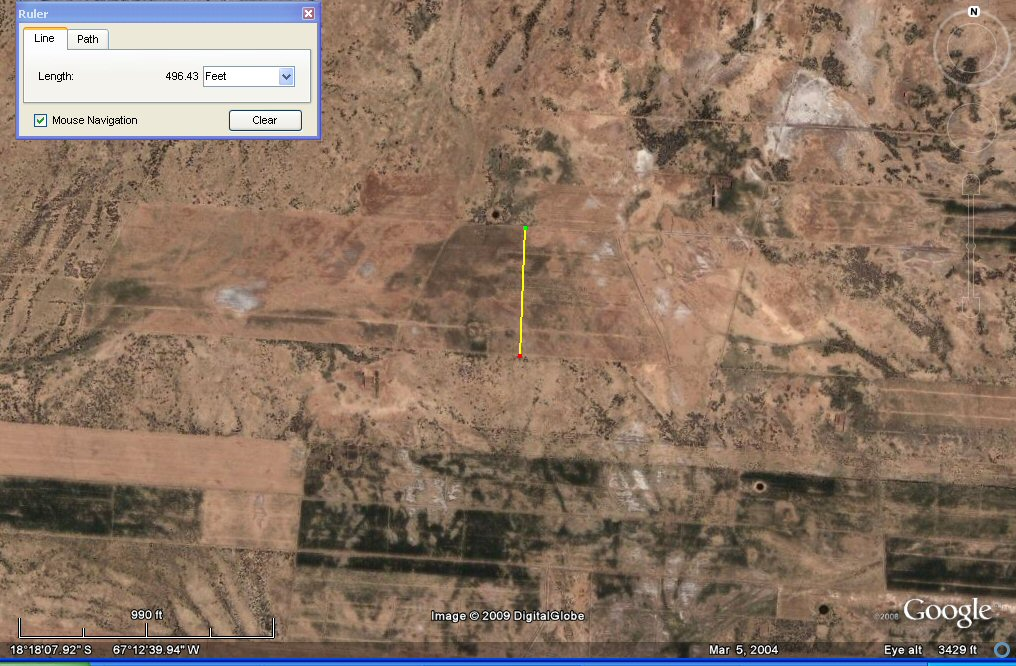above, image 40, intended distance 495 feet is 300 cubits of 19.8"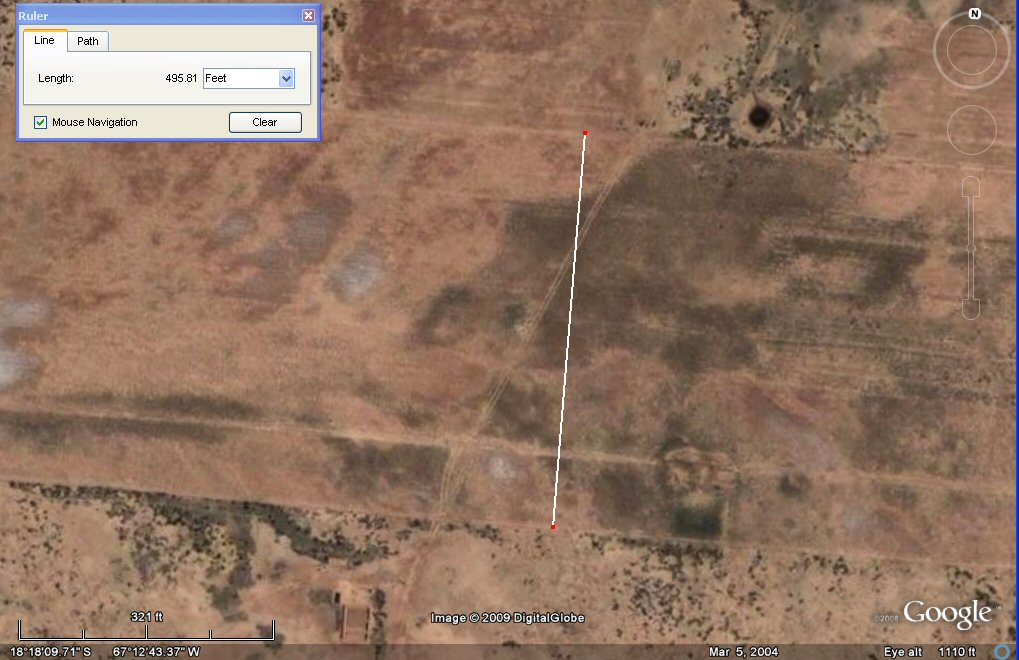above, image 40b, closer magnification gives more accurate measurement, intended distance 495 feet is 300 cubits of 19.8"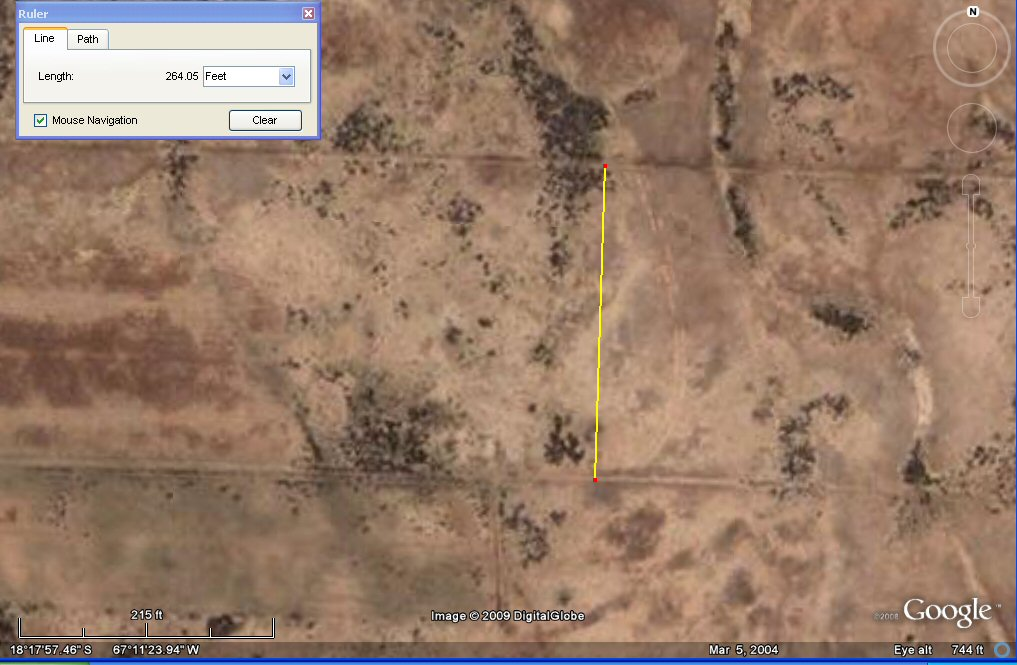above, image 44, close magnification gives more accurate measurement, intended distance 264 feet is 160 cubits of 19.8"

Teotihuacan measuring unit

Teotihuacan measuring unit at the citadel

Chichen Itza and El Castillo cubits